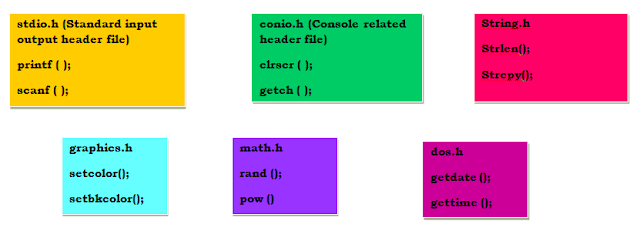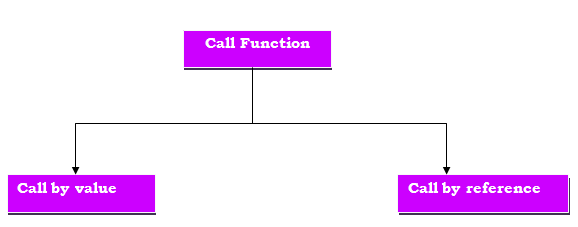## Monday, 25 February 2019

### The need for C functions

When we write programs, sometimes it happens that we have to type any code repeatedly, so to avoid this situation, we create C functions. Creating function reduces the consumption of memory space and we can use that code in the program when needed.

With the help of the function, we divide a large program into smaller parts, which also facilitates debugging the program.

### What are C functions?

C functions are a group of many codes/instructions and statements that work together to execute a task.

### Categorization of C functions

1. Library function or pre-defined function
2. User-defined function

#### 1. Library function

The library function is a pre-defined function in C library. Library function includes header files like stdio.h, conio.h, string.h, graphics.h, dos.h, math.h etc. All header files we can include in our program using "#include <filename.h>" and these header files include C functions.Library functions in C

#### 2. User-defined function

These functions are made by themselves. You can also modify these functions. We will further understand how to create user-defined functions, but before that how do we create functions.

### Function declaration

A function declaration is a process in which it tells the compiler about the function name, it's return type and parameter list. It is also called function prototyping.

Syntax

return type         name_of_function (parameter list);

return type- return type tells the compiler the value you want to return is of which data type (int, float, double, char).
Function name- Function name is an identifier which is decided by the programmer.
Parameter list- This list declares the data type and arguments at the time of calling the function.

If we write declarations before the main function, then this prototype is called a global prototype and this declaration is available for all the functions written in the program.

When we write the declaration in the function definition then the prototype is called the local prototype.

### Function definition

A function definition is a group of statements which are written in the function body.

Syntax

return type     name_of_function (parameter_list)
{
Function body;
}

### Calling function

When we call a function, the control goes to the function body.

Syntax

Function_name (argument_list);

### A c program which demonstrates the function.

#include <stdio.h>

// Declaration of addition function
int add_function(int a, int b);
int main ()
{
int c;
//Calling of addition function
printf ("c=%d", c);
return 0;
}

//Function definition
int add_function(int a, int b)
{
}

Output
c=30

### Call C function in a programCall C function

### Call by value

#include <stdio.h>
//Declaration of maximum function
int maximun(int a, int b);
int main ()
{
int a = 11, b=21,c;
//Calling maximum function
c = maximum(a,b);
printf ("c=%d\n",c);
return 0;
}

//Maximum function definition
int maximum(int a, int b)
{
int temp;
if (a>b)
{
temp=a;
}
else
{
temp=b;
}
return temp;
}

Explanation

In this function, we declare the value of the variable 'a' and variable 'b' in the main function, these values stored in the stack memory. If we want to redeclare these values in the local function (maximum), no changes in the values of the local function. values of the variable in the main function will not change.

Output
c = 21

### Call by reference

#include <stdio.h>
// Declaration of function of swapping two numbers
int swap (int *a, int *b);
int main()
{
int x = 19, y=56;
// Calling function swap
swap(&x,&y);
printf ("x=%d\n y=%d\n", x,y);
return 0;
}

//Function Definition of swap
int swap(int *a, int *b)
{
int temp;
temp = *a;
*a = *b;
*b = temp;
}

Explanation

In call by reference, the address of variables is assigned to the function as a parameter. We can change the value of the variables in the local function (swap).  Same memory is used for variables declared in the main function or if you want to redeclare variable in the local function.

Output

x = 56
y = 19